# NCERT Solutions for Class 10 Science Chapter 11 - Magnetic Effects of Electric Current

##### Question 1:

Why does a compass needle get deflected when brought near a bar magnet?

A compass needle is a small bar magnet with one north and the other south pole. When a magnet is brought near the compass, the north pole of a compass gets attracted by the south pole of the magnet and hence, gets deflected.

##### Question 2:

Draw magnetic field lines around a bar magnet.

Take a small compass and a bar magnet. Place the bar magnet on a sheet of a paper fixed on a drawing board. Place the compass near the north pole of the magnet. The south pole of compass will point towards the north pole of the magnet. Mark the two points a, b in the direction of south pole and north pole of the needle. Move the needle to a new position such that S of compass is at b [position previously held by N. Proceed till you reach the south pole of the magnet. Join the marked points by a smooth line which gives one magnetic line of force. Repeat the procedure taking different starting points.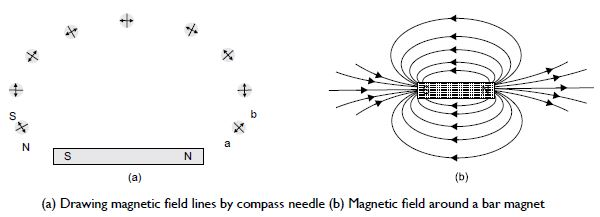##### Question 3:

List the properties of magnetic field lines.

The properties of magnetic lines of force are:
1. Outside the magnet, they emerge from the north pole of the magnet and enter the south pole.
2. The two magnetic lines of force never intersect each other.
3. Inside the magnet, these lines are directed from the south to the north pole.
4. They have a tendency to contract lengthwise. This explains the attraction between the opposite poles.
5. They exert lateral pressure upon each other. This explains repulsion between the like poles.

##### Question 4:

Why don’t two magnetic field lines intersect each other?

The tangent to a field line gives the direction of magnetic field at that point. No two magnetic lines of force cross each other because if they did so, it will mean that at the point of intersection, the compass needle would point towards two directions which is not possible. Hence, two magnetic field lines never intersect each other.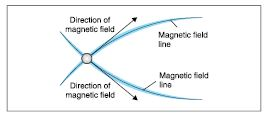##### Question 5:

Consider a circular loop of wire lying in the plane of the table. Let the current pass through the loop clockwise. Apply the right-hand rule to find out the direction of the magnetic field inside and outside the loop.

The direction of the magnetic field inside and outside the loop is as shown in [figure. (a)]. Magnetic field inside the loop is perpendicular to the table top in downward direction [figure. (b)]. Magnetic field outside the loop is perpendicular to the table top in upward direction [figure. (c)].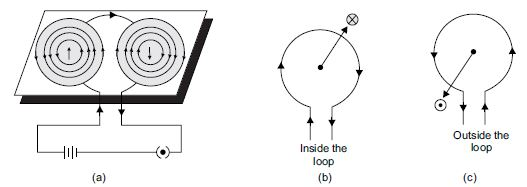##### Question 6:

The magnetic field in a given region is uniform. Draw a diagram to represent it.

Uniform magnetic field is represented by equidistant and parallel lines as shown in the figure. The parallel lines are close to each other if the field is strong.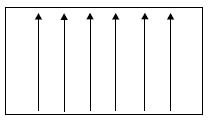##### Question 7:

Choose the correct option.
The magnetic field inside a long straight solenoid carrying current

1. is zero
2. decreases as we move towards the end
3. increases as we move towards end
4. is same at all the points

(d) is correct. The magnetic field inside a long straight current-carrying solenoid is uniform which is represented by parallel lines. Hence, it is same at all the points.

##### Question 8:

Which of the following property of proton can change while it moves freely in a magnetic field?
[There may be more than one correct answer].

1. mass
2. speed
3. velocity
4. momentum

(c) and (d) are correct. Velocity and momentum of proton can change while it moves freely in a magnetic field.

##### Question 9:

In the activity shown below, how do you think the displacement of rod AB will be affected if
(i) the current in the rod AB is increased
(ii) a stronger horse-shoe magnet is used
(iii) the length of the rod AB is increased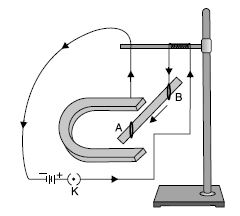(i) Since the force acting on the rod is directly proportional to the current passing through it, therefore, the displacement will be increased when the current is increased.
(ii) The stronger the magnet, the more will be force and hence, more will be the displacement.
(iii) Force is directly proportional to the length of the rod. Hence, the rod will be displaced more if the length is increased.

##### Question 10:

A positively charged particle (α-particle) projected towards west is deflected towards north by a magnetic field. The direction of the magnetic field is

1. towards south
2. towards east
3. downward
4. upward

(d) Here, the direction of positively charged alpha particle is towards the west, i.e., the direction of current is also towards the west and the direction of magnetic force is towards north. Hence, according to Fleming’s left-hand rule, the direction of magnetic field will be upwards.

##### Question 11:

State Fleming’s left-hand rule.

Fleming’s left-hand rule: Stretch the thumb, forefinger and middle finger of your left hand such that they are mutually perpendicular to each other. If the first finger points in the direction of magnetic field and central (second) finger points towards the direction of current then the thumb points towards the direction of force or towards motion as shown in the figure.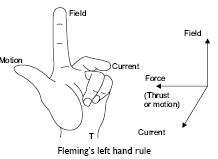##### Question 12:

What is the principle of an electric motor?

When a current carrying coil is placed in a magnetic field, it experiences a torque. Due to this torque, the coil begins to rotate.

##### Question 13:

What is the role of the split ring in an electric motor?

The split ring acts as a commutator in a DC motor i.e., as a device which reverses the direction of current through the circuit after every half cycle.

##### Question 14:

Explain the different ways to induce current in a coil.

There are mainly two methods to induce the current in a coil.
1. By relative motion between the coil and a magnet: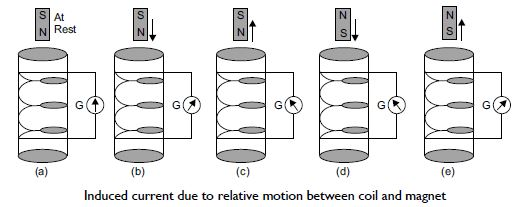When the magnet is stationary, i.e., at rest as in [figure (a)], the induced current is not produced. When magnet is moving with N-pole towards the coil, deflection is as shown in [figure (b)]. The direction of the current gets reversed when the N-pole moves away from the coil as shown in [figure (c)]. The faster the magnet moves, the more the deflection and hence, more current in the galvanometer.
The deflection produced by the current is same when N-pole is moving towards the coil [figure (b)] or when the S-pole is moving away from the coil [figure (e)]. The current and hence deflection in the galvanometer will be produced again if the magnet is kept at rest and the coil is moved.
2. By changing current in the neighbouring circuit: Take a non-conducting cylindrical tube made from a thick paper. Wind two set of coils 1 and 2 on it as shown. Connect a battery and a key to coil I and a galvanometer to coil 2. When a key is inserted in K, there will be an instantaneous deflection in the galvanometer connected to coil 2 even though there is no cell connected to coil 2. Take out the key from plug K. An instantaneous large deflection in a direction opposite to the previous direction will be produced in the galvanometer. Thus, current has been induced in coil 2 due to the increase or decrease of current in coil I.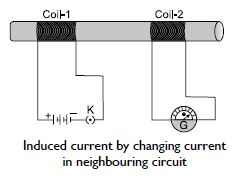##### Question 15:

State the principle of electric generator.

Electric generator is based upon the principle of electromagnetic induction. Principle: When a coil is rotated in a magnetic field, a current will be induced. This current is known as induced current.

##### Question 16:

Name some sources of direct current.

Sources of pure direct current are cell, battery etc.

##### Question 17:

Which sources produce alternating current?

Alternating current is produced by AC generators. There are hydro-generator and thermal generators. Hydro-generators convert potential energy of water to electricity whereas thermal generators convert heat energy of the steam to electric energy.

##### Question 18:

Choose the correct option.
A rectangular coil of copper wires is rotated in a magnetic field. The direction of induced current changes once in each

1. two revolutions
2. one revolution
3. half revolution
4. one-fourth revolution

half revolution

##### Question 19:

Name two safety measures commonly used in electric circuits and appliances.

Two safety measures used are:
1. A safety fuse of proper rating: A fuse in a circuit which prevents damage to the appliances and circuit due to overloading or short circuiting.
2. An earth wire: It prevents possible shock when live wire accidentally touches the body of an appliance.

##### Question 20:

An electric oven of 2 kW power rating is operated in a domestic electric circuit (220 V) that has a current rating of 5 A. What result do you expect? Explain.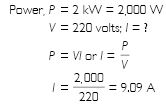A current of 9.09 A will flow in the circuit. Since the current rating of circuit is 5 A, the fuse of 5 A rating if inserted in the circuit will burn up. If no fuse has been put in the circuit, there may be a fire.

##### Question 21:

What precaution should be taken to avoid the overloading of domestic electric circuits?

1. Wires used for carrying current should be of proper current rating.
2. Wires of low current rating may be used for lighting electric bulbs, tubes, TV etc., wires of higher current rating should be used for AC, heating appliances etc.
3. Each circuit should have a fuse of proper rating.
4. Wire should be replaced by new wires of proper rating after every 3–4 years.
5. PVC of good quality should be used.

##### Question 22:

Which of the following correctly describes the magnetic field near a long straight wire? The field consists of
(a) straight lines perpendicular to the wire
(b) straight lines parallel to the wire
(c) radial lines originating from the wire
(d) concentric circles centered on the wire

(d) concentric circles centered on the wire as shown in figures (a) and (b)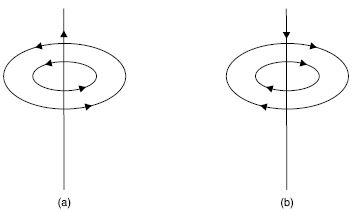##### Question 23:

The phenomenon of electromagnetic induction is

1. the process of charging a body
2. the process of generating magnetic field due to a current passing through a coil
3. producing induced current in a coil due to relative motion between a magnet and the coil
4. the process of rotating a coil of an electric motor

producing induced current in a coil due to relative motion between a magnet and the coil

##### Question 24:

The device used for producing electric current is called

1. generator
2. galvanometer
3. ammeter
4. motor

generator

##### Question 25:

The essential difference between an AC generator and a DC generator is that

1. AC generator has an electromagnet while a DC generator has a permanent magnet
2. DC generator will generate higher voltage
3. AC generator will generate higher voltage/li>
4. AC generator has slip rings while the DC generator has a commutator

AC generator has slip rings while the DC generator has a commutator

##### Question 26:

At the time of short circuit, the current in the circuit

1. reduces substantially
2. does not change
3. increases heavily
4. vary continuously

increases heavily

##### Question 27:

State whether the following statements are true or false: An electric motor converts mechanical energy into electric energy.

FALSE

##### Question 28:

State whether the following statements are true or false: An electric generator works on the principle of electromagnetic induction.

TRUE

##### Question 29:

State whether the following statements are true or false: The field at the centre of a long circular coil carrying current will be parallel straight lines.

TRUE

##### Question 30:

State whether the following statements are true or false:
A wire with a green insulation is usually the live wire of an electric supply.

FALSE

##### Question 31:

List three sources of magnetic field.

The three sources are:
1. magnet
2. current-carrying conductor
3. current-carrying solenoid

.
##### Question 32:

How does a solenoid behave like a magnet? Can you determine the north and south poles of a current-carrying solenoid with the help of a bar magnet? Explain.

Solenoid is a coil of a number of turns of insulated copper wire closely wrapped in the shape of a cylinder. Magnetic field around a current carrying solenoid is shown in figure (a).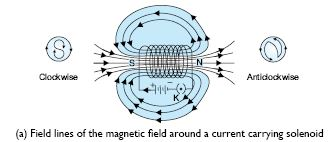These appear to be similar to that of a bar magnet shown in figure (b).
The poles of the solenoid can be determined using the clock rule. While looking from one end of the coil, if the current is in the clockwise direction, that end is the south pole, while if the current is in the anticlockwise direction the end is the north pole. Here, the right end of the solenoid is the north pole whereas the left end behaves as the south pole.
Magnetic field lines inside the solenoid are in the form of parallel straight lines. This means that the field is the same at all points inside the solenoid. When a soft iron rod is placed inside the solenoid, it behaves like an electromagnet.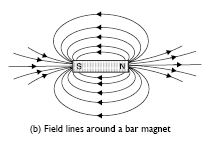##### Question 33:

How does a solenoid behave like a magnet? Can you determine the north and south poles of a current-carrying solenoid with the help of a bar magnet? Explain.

Solenoid is a coil of a number of turns of insulated copper wire closely wrapped in the shape of a cylinder. Magnetic field around a current carrying solenoid is shown in figure (a).These appear to be similar to that of a bar magnet shown in figure (b). The poles of the solenoid can be determined using the clock rule. While looking from one end of the coil, if the current is in the clockwise direction, that end is the south pole, while if the current is in the anticlockwise direction the end is the north pole. Here, the right end of the solenoid is the north pole whereas the left end behaves as the south pole.
Magnetic field lines inside the solenoid are in the form of parallel straight lines. This means that the field is the same at all points inside the solenoid. When a soft iron rod is placed inside the solenoid, it behaves like an electromagnet.##### Question 34:

When is the force experienced by a current-carrying conductor, placed in a magnetic field, the largest?

From Fleming’s left-hand rule, the force experienced by a current carrying conductor placed in a magnetic field is maximum (largest) when the direction of field is perpendicular to the current-carrying conductor.

##### Question 35:

Imagine that you are sitting in a chamber with your back to one wall. An electron beam moving horizontally from the back wall towards the front wall is deflected by a strong magnetic field to your right side. What is the direction of the magnetic field?

The magnetic field will be acting in a vertically downward direction in accordance with Fleming’s left-hand rule. The direction of the current should be considered in a direction opposite to the direction of motion of electrons.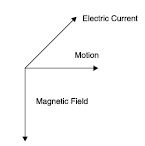##### Question 36:

Draw a labelled diagram of an electric motor. Explain its principle and working. What is the function of a split ring in an electric motor?

Principle: When a current-carrying conductor capable of free movement is placed in a magnetic field, it experiences a mechanical force and begins to move in a direction given by Fleming’s left-hand rule.
Construction: A DC motor consists of a single coil ABCD called armature between the pole pieces of a magnet as shown in the figure. Armature consists of a coil of a large number of turns of insulated wire wrapped on a soft iron core.
The two ends of the armature are connected to segments S1 and S2 of a commutator. The brushes B1 and B2 keep their contact with the commutator as it rotates. A battery is connected across the brushes. Working: A direct current from a battery is passed to the armature. The current flows in the coil along ABCD as shown in figure (a). The arm AB of the coil experience downward force and CD of the coil experience upward force in accordance with Fleming’s left-hand rule. These two equal and opposite forces constitute a couple tending to rotate the coil in anticlockwise direction. After half rotation, brush B1 comes in contact with S2 and brush B2 with S1. The direction of the current gets reversed. Commutator or split rings help in reversing the current in the coil after every half rotation. The current now flows along DCBA instead of ABCD. Arm DC experiences downward force and BA experiences an upward force in accordance with Fleming’s left-hand rule. The reversing of current is repeated at each half rotation, giving rise to a continuous rotation of the coil.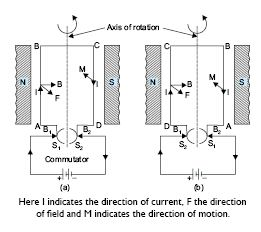##### Question 37:

Name some devices in which electric motors are used.

Electric motors are used in battery-operated toys, tape recorder, car fans and a variety of other electric appliances.

##### Question 38:

A coil of insulated copper wire is connected to a galvanometer. What will happen if a bar magnet is
(i) pushed into the coil;
(ii) withdrawn from inside the coil;
(iii) held stationary inside the coil?

When the bar magnet is pushed into the coil, there will be a momentary deflection in one direction. The faster we push the magnet, the more will be the deflection.
When the bar magnet is withdrawn, there will be again a momentary deflection but in a direction opposite to that when the magnet was pushed into the coil.
When the magnet is held stationary in the coil, there will be no deflection in the galvanometer.

##### Question 39:

Two circular coils A and B are placed close to each other. If the current in the coil A is changed, will some current be induced in the coil B? Give reason.

If the current in the coil A is changed then some current will be induced in the coil B. When the current in coil A is changed, the magnetic field associated with it also changes. As a result, the magnetic field around coil B also changes. This change in magnetic field lines around the coil B induces an electric current in it.

##### Question 40:

State the rule to determine the direction of (i) a magnetic field produced around a straight conductor carrying current; (ii) force experienced by a current carrying straight conductor placed in a magnetic field which is perpendicular to it, and (iii) current induced in a coil due to its rotation in a magnetic field.

(i) The right-hand thumb rule will determine the direction of a magnetic field around a straight conductor carrying current.
(ii) Fleming’s left-hand rule will determine the direction of the force experienced by a current carrying straight conductor placed in a magnetic field.
(iii) Fleming’s right-hand rule will determine the direction of current induced in a coil due to its rotation in a magnetic field.

##### Question 41:

Explain the underlying principle and working of an electric generator by drawing a labelled diagram. What is the function of brushes?

Principle: Alternators are based on the principle that an induced current is produced in a conductor whenever there is change in magnetic lines of force linked with it. The direction of induced current is given by Fleming’s right-hand rule stated below.
Fleming’s right-hand rule: If the thumb, forefinger and central finger of right hand are stretched out mutually at right angles to each other such that the forefinger points along the direction of magnetic field and thumb along the direction of motion of the conductor, then the central finger indicates the direction of the current produced in the coil. Construction: A simple AC generator consists of the following important parts:
(i) Armature: It consists of a coil ABCD having a large number of insulated copper wires wound over a soft iron core. This coil is called armature. The armature is mounted on an axle which can be rotated by the force exerted by falling water, wind or steam.
(ii) Field magnets: The coil is held between the pole pieces of a strong magnet called the field magnet. In small dynamos, the magnetic field is provided by a permanent magnet.
(iii) Slip rings: These consist of two hollow metallic rings R1 and R2 mounted on the axle of the coil. The ends of the arms AB and CD of the coil are connected to the rings R1 and R2 respectively. These rings rotate along with the rotation of the armature.
(iv) Current from B1, B2 is taken out to the load connected across two brushes.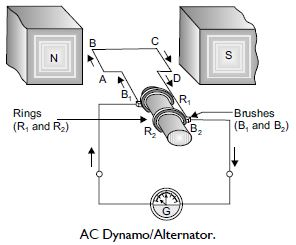Working: Let the coil initially be in a horizontal position as shown in the figure. Let the coil be rotated in a clockwise direction between the pole pieces N and S of a horse-shoe magnet.
At an instant, let AB moves up and CD move down as shown in the figure. Using Fleming’s right-hand rule to the sides AB and DC of the coil, we observe that the current flows in the direction A to B and from C to D. Effective current flows along ABCD.
After half rotation, sides AB and DC of the coil will interchange their positions. Now CD starts moving up and AB downwards. The direction of induced current in each side is reversed after half rotation. Due to the change in the direction of induced current in the coil after half rotation, positive or negative polarity of the two ends is also changed.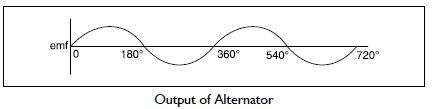##### Question 42:

When does an electric short circuit occur?

Electric short circuit occurs when:
(i) live wire accidentally touches neutral wire.
(ii) insulation around the current-carrying wires is weak.
(iii) insulation gets hardened by excessive use.
(iv) current passed through the wire is more than its fuse rating.

##### Question 43:

What is the function of an earth wire? Why is it necessary to earth metallic appliances?